Courses

# Notes | EduRev

## Class 10 : Notes | EduRev

The document Notes | EduRev is a part of the Class 10 Course Class 10 Mathematics by VP Classes.
All you need of Class 10 at this link: Class 10

Q 15. Five cards—the ten, jack, queen, king and ace of diamonds, are well-shuffled with their face downwards. One card is then picked up at random.
(i) What is the probability that the card is the queen?
(ii) If the queen is drawn and put aside, what is the probability that the second card picked up is (a) an ace? and (b) a queen?
Sol. We have five cards.
∴ All possible outcomes = 5
(i) For a queen:
∵ Number of queens = 1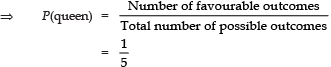(ii) The queen is drawn and put aside,
∴ Only 5 − 1 = 4 cards are left,
⇒ All possible outcomes = 4
(a) For an ace:
∵ There is only one ace
∴ Number favourable outcomes = 1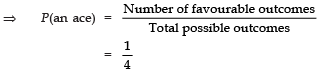(b) For a queen:
Since, the only queen has already been put aside.
∴ Number of possible outcomes = 0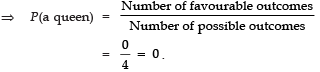Q 16. 12 defective pens are accidentally mixed with 132 good ones. It is not possible to just look at a pen and tell whether or not it is defective. One pen is taken out at random from this lot. Determine the probability that the pen taken out is a good one.
Sol. We have
Number of good pens = 132
Number of defective pens = 1 2
∴ Total number of pens = 132 + 12 = 144
For good pens:
∵ There are 132 good pens
∴ Number of favourable outcomes = 132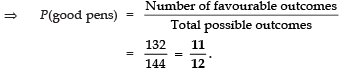Q 17. (i) A lot of 20 bulbs contain 4 defective ones. One bulb is drawn at random from the lot. What is the probability that this bulb is defective?
(ii) Suppose the bulb drawn in (i) is not defective and is not replaced. Now one bulb is drawn at random from the rest. What is the probability that this bulb is not defective?
Sol. Since, there are 20 bulbs in the lot.
∴ Total number of possible outcomes = 20
(i) ∵ Number defective bulbs = 4
i.e., Favourable outcomes = 4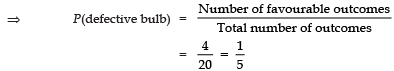(ii) ∵ The bulb drawn above is not included in the lot.
∴ Remaining number of bulbs = 20 − 1 = 19.
⇒ Total number of possible outcomes = 19.
∵ Number of bulbs which are not defective = 19 − 4 = 15
⇒ Favourable number of outcomes = 15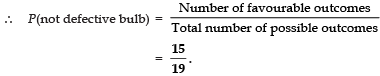Q 18. A box contains 90 discs which are numbered from 1 to 90. If one disc is drawn at random from the box, find the probability that it bears (i) a two-digit number, (ii) a perfect square number and (iii) a number divisible by 5.

Sol. We have:
Total number of discs = 90
∴ Total number of possible outcomes = 90
(i) For a two-digit number:
Since the two-digit numbers are 10, 11, 12, ....., 90.
∴ Number of two-digit numbers = 90 − 9 = 81
[∵ 1, 2, 3, 4, 5, 6, 7, 8, and 9 are 1-digit numbers]
⇒ Number of favourable outcomes = 81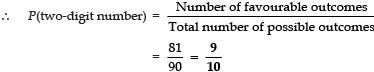(ii) For a perfect square:
Perfect squares from 1 to 90 are 1, 4, 9, 16, 25, 36, 49, 64, and 81
∴ Number of perfect numbers = 9
⇒ Number of favourable outcomes = 9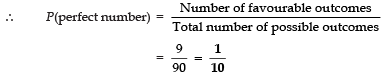(iii) For a number divisible by 5:
Numbers divisible by 5 [from 1 to 90] are: 5, 10, 15, 20, 25, 30, 35, 40, 45, 50, 55, 60, 65, 70, 75, 80, 85, 90
i.e. There are 18 number (1 to 90) which are divisible by 5.
∴ Number of favourable outcomes = 18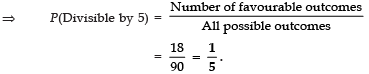Q 19. A child has a die whose six faces show the letters as given below: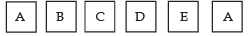The die is thrown once. What is the probability of getting (i) A? and (ii) D?
Sol. Since there are six faces of the given die and these faces are marked with letters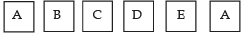∴ Total number of letters = 6
⇒ Number of possible outcomes = 6
(i) For the letter A
∵ Two faces are having the letter A.
∴ Number of favourable outcomes = 2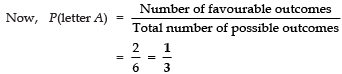(ii) For the letter D:
∵ Number of D’s = 1
∴ Number of possible outcomes = 1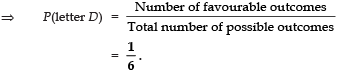Q 20. Suppose you drop a die at random on the rectangular region shown in figure. What is the probability that it will land inside the circle with diameter 1 m?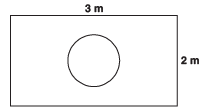Sol. Here, Area of the rectangle = 3 m × 2 m = 6 m2
And, the area of the circle = πr2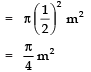∴ Probability for the die to fall inside the circle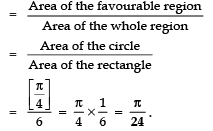Q 21. A lot consists of 144 ball pens of which 20 are defective and the others are good. Nuri will buy a pen if it is good, but will not buy if it is defective. The shopkeeper draws one pen at random and gives it to her. What is the probability that (i) She will buy it? (ii) She will not buy it?
Sol. Total number of ball pens = 144
⇒ All possible outcomes = 144
(i) Since there are 20 defective pens
∴ Number of good pens 144 − 20 = 124
⇒ Number of favourable outcomes = 124
∴ Probability that she will buy it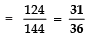(ii) Probability that she will not buy it
=1 − [Probability that she will buy it]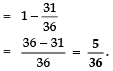Q 22. Two dice one blue and one grey, are thrown at the same time. Now Complete the following table: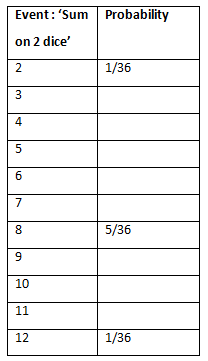(ii) A student argues that there are 11 possible outcomes 2, 3, 4, 5, 6, 7, 8, 9, 10, 11 and 12. Therefore, each of them has a probability 1/11. Do you agree with this argument? Justify your answer.
Sol. ∵ The two dice are thrown together.
∴ Following are the possible outcomes:
(1, 1); (1, 2); (1, 3); (1, 4); (1, 5); (1, 6).
(2, 1); (2, 2); (2, 3); (2, 4); (2, 5); (2, 6).
(3, 1); (3, 2); (3, 3); (3, 4); (3, 5); (3, 6).
(4, 1); (4, 2); (4, 3); (4, 4); (4, 5); (4, 6).
(5, 1); (5, 2); (5, 3); (5, 4); (5, 5); (5, 6).
(6, 1); (6, 2); (6, 3); (6, 4); (6, 5); (6, 6).
⇒ Number of all possible outcomes is 6 × 6 = 36.
(i) Let the required probability be P(E).
(a) ∵ The sum on two dice is 3 for: (1, 2) and (2, 1)
∴ Favourable outcomes = 2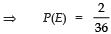(b) a The sum on two dice is 4 for: (1, 3), (2, 2) and (3, 1).
∴ Number of favourable outcomes = 3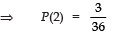(c) ∵ The sum on two dice is 5 for:
(1, 4), (2, 3), (3, 2) and (4, 1)
∴ Number of favourable outcomes = 4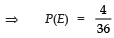(d) The sum on two dice is 6 for:
(1, 5), (2, 4), (3, 3), (4, 2) and (5, 1)
∴ Number favourable outcomes = 5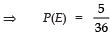(e) The sum on two dice is 7 for:
(1, 6), (2, 5), (3, 4), (4, 3), (5, 2) and (6, 1)
∴ Number of favourable outcomes = 6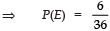(f) The sum on two dice is 9 for:
(3, 6), (4, 5), (5, 4) and (6, 3)
∴ Number of favourable outcome = 4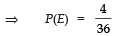(g) The sum on two dice is 10 for:
(4, 6), (5, 5), (6, 4)
∴ Number of favourable outcomes = 3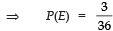(h) The sum on two dice is 11 for:
(5, 6) and (6, 5)
∴ Number of favourable outcomes = 2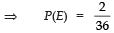Thus, the complete table is as under: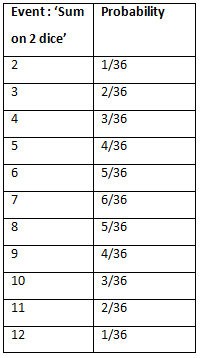(ii) No. The number of all possible outcomes is 36 and not 11.
∴ The argument is not correct.

Q 23. A game consists of tossing a one rupee coin 3 times and noting its outcome each time. Hanif wins if all the tosses give the same result i.e., three heads or three tails, and loses otherwise. Calculate the probability that Hanif will lose the game.
Sol. Let T denotes the tail and H denotes the head.
∴ All the possible outcomes are:
HHH, HHT, HTT, TTT, TTH, THT, TTH, HTH
∴ Number of all possible outcomes = 8
Let the event that Hanif will lose the game be denoted by E.
∴ Favourable events are:
HHT, HTH, THH, THT , TTH, HTT
⇒ Number of favourable outcomes = 3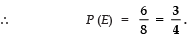Q 24. A die is thrown twice. What is the probability that
(i) 5 will not come up either time?
(ii) 5 will come up at least once?
[Hint: Throwing a die twice and throwing two dice simultaneously are treated as the same experiment.]
Sol. Since, throwing a die twice or throwing two dice simultaneously is the same.
∴ All possible outcomes are:
(1, 1); (1, 2); (1, 3); (1, 4); (1, 5); (1, 6)
(2, 1); (2, 2); (2, 3); (2, 4); (2, 5); (2, 6)
(3, 1); (3, 2); (3, 3); (3, 4); (3, 5); (3, 6)
(4, 1); (4, 2); (4, 3); (4, 4); (4, 5); (4, 6)
(5, 1); (5, 2); (5, 3); (5, 4); (5, 5); (5, 6)
(6, 1); (6, 2); (6, 3); (6, 4); (6, 5); (6, 6)
∴ All possible outcomes = 36
(i) Let E be the event that 5 does not come up either time, then
The favourable outcomes are [36 − (5 + 6)] = 25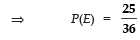(ii) Let N be the event that 5 will come up at least once, then Number of favourable outcomes = 5 + 6 = 11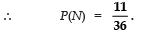Q 25. Which of the following arguments are correct and which are not correct? Give reasons for your answer. (i) If two coins are tossed simultaneously there are three possible outcomes—two heads, two tails or one of each. Therefore, for each of these outcomes, the probability is 1/3.
(ii) If a die is thrown, there are two possible outcomes—an odd number or an even number.
Therefore, the probability of getting an odd number is 1/2.

Sol. (i) Argument is incorrect.
The possible outcomes are- (HH), (HT), (TH), (TT)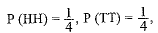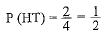(ii) Argument is correct.
Possible outcomes = 1, 2, 3, 4, 5, 6
Odd numbers are = 1, 3, 5,
Hence P (an odd number) = 3/6 = 1/2
Even numbers are = 2, 4, 6,
Hence P (an even number) = 3/6 = 1/2

Offer running on EduRev: Apply code STAYHOME200 to get INR 200 off on our premium plan EduRev Infinity!

132 docs

,

,

,

,

,

,

,

,

,

,

,

,

,

,

,

,

,

,

,

,

,

;